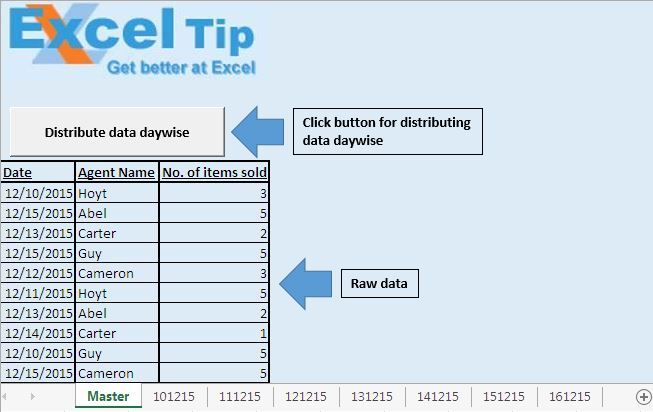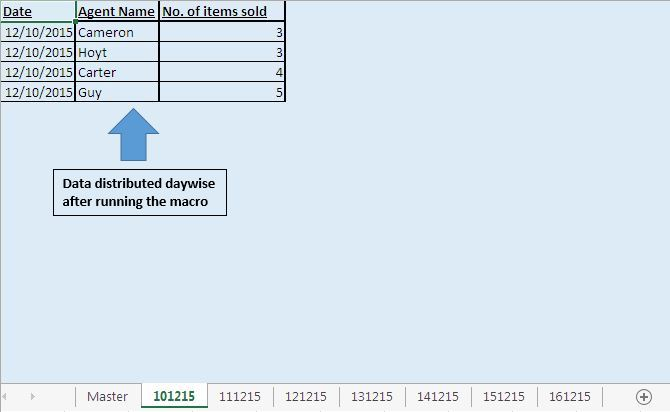# Distribute Data from a List of the day to day sheets using VBA

In this article, we will create a macro to arrange data day-wise on multiple sheets.

Raw data for this article consists day-wise sales data of the team, which includes Date, Agent Name and Number of items sold.We want to distribute the data day-wise on multiple sheets. Clicking on the “Distribute data daywise” button, will do the same.Code explanation

Do Until IsEmpty(Worksheets(1).Cells(intRowS, 1))

Loop

In above code, Do Until loop will loop until a blank cell is encountered.

strTab = Format(Cells(intRowS, 1).Value, "ddmmyy")

The above code is used to extract sheet name from the date.

intRowT = Worksheets(strTab).Cells(Rows.Count, 1).End(xlUp).Row + 1

The above code is used to get row number of the last cell.

```Sub Divide()

'Declaring variables
Dim intRowS As Integer, intRowT As Integer
Dim strTab As String

'Initializing with starting row number
intRowS = 10

'Checking whether cell in first column is empty
Do Until IsEmpty(Worksheets(1).Cells(intRowS, 1))

'Getting name of the sheet based on the date value in the first column
strTab = Format(Cells(intRowS, 1).Value, "ddmmyy")

'Getting the row number of last cell
intRowT = Worksheets(strTab).Cells(Rows.Count, 1).End(xlUp).Row + 1

'Copying data to respective sheet
Rows(intRowS).Copy Worksheets(strTab).Rows(intRowT)
intRowS = intRowS + 1
Loop

End Sub
```